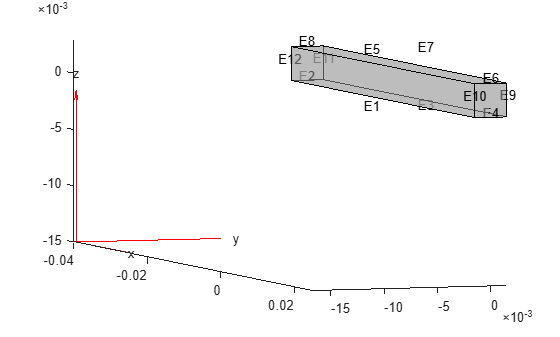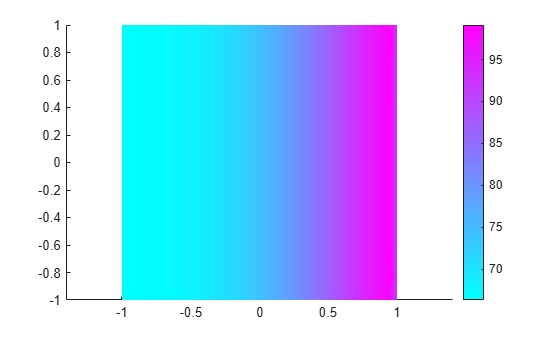# reconstructSolution

Recover full-model transient solution from reduced-order model (ROM)

## Syntax

``structuralresults = reconstructSolution(Rcb,u,ut,utt,tlist)``
``thermalresults = reconstructSolution(Rtherm,u_therm,tlist)``

## Description

example

````structuralresults = reconstructSolution(Rcb,u,ut,utt,tlist)` recovers the full transient structural solution from the reduced-order model `Rcb`, displacement `u`, velocity `ut`, and acceleration `utt`. Typically, the displacement, velocity, and acceleration are the values returned by Simscape™.```

example

````thermalresults = reconstructSolution(Rtherm,u_therm,tlist)` recovers the full transient thermal solution from the reduced-order model `Rtherm`, temperature in modal coordinates `u_therm`, and the time-steps `tlist` that you used to solve the reduced model.```

## Examples

collapse all

Knowing the solution in terms of the interface degrees of freedom (DoFs) and modal DoFs, reconstruct the solution for the full structural transient model.

Create a structural model for transient analysis.

`modelT = createpde('structural','transient-solid');`

Create a square cross-section beam geometry and include it in the model.

```gm = multicuboid(0.05,0.003,0.003); modelT.Geometry = gm;```

Plot the geometry, displaying face and edge labels.

```figure pdegplot(modelT,'FaceLabels','on','FaceAlpha',0.5) view([71 4])``````figure pdegplot(modelT,'EdgeLabels','on','FaceAlpha',0.5) view([71 4])```Specify Young's modulus, Poisson's ratio, and the mass density of the material.

```structuralProperties(modelT,'YoungsModulus',210E9, ... 'PoissonsRatio',0.3, ... 'MassDensity',7800);```

Fix one end of the beam.

`structuralBC(modelT,'Edge',[2 8 11 12],'Constraint','fixed');`

Add a vertex at the center of face 3.

`loadedVertex = addVertex(gm,'Coordinates',[0.025 0.0 0.0015]);`

Generate a mesh.

`generateMesh(modelT);`

Apply a sinusoidal concentrated force in the z-direction on the new vertex.

```structuralBoundaryLoad(modelT,'Vertex',loadedVertex, ... 'Force',[0;0;10],'Frequency',6000);```

Specify zero initial conditions.

`structuralIC(modelT,'Velocity',[0 0 0],'Displacement',[0 0 0]);`

Define superelement interfaces using the fixed and loaded boundaries. In this case, the reduced-order model retains the DoFs on the fixed face and the loaded vertex while condensing all other DoFs in favor of modal DoFs. For better performance, use the set of edges bounding face 5 instead of using the entire face.

```structuralSEInterface(modelT,'Edge',[2 8 11 12]); structuralSEInterface(modelT,'Vertex',loadedVertex);```

Reduce the structure, retaining all fixed interface modes up to `5e5`.

`rom = reduce(modelT,'FrequencyRange',[-0.1,5e5]);`

Next, use the reduced-order model to simulate the transient dynamics. Use the `ode15s` function directly to integrate the reduced system ODE. Working with the reduced model requires indexing into the reduced system matrices `rom.K` and `rom.M`. First, construct mappings of indices of `K` and `M` to loaded and fixed DoFs by using the data available in `rom`.

DoFs correspond to translational displacements. If the number of mesh points in a model is `Nn`, then the toolbox assigns the IDs to the DoFs as follows: the first `1` to `Nn` are x-displacements, `Nn+1` to `2*Nn` are y-displacements, and `2Nn+1` to `3*Nn` are z-displacements. The reduced model object `rom` contains these IDs for the retained DoFs in `rom.RetainedDoF`.

Create a function that returns DoF IDs given node IDs and the number of nodes.

`getDoF = @(x,numNodes) [x(:); x(:) + numNodes; x(:) + 2*numNodes];`

Knowing the DoF IDs for the given node IDs, use the `intersect` function to find the required indices.

```numNodes = size(rom.Mesh.Nodes,2); loadedNode = findNodes(rom.Mesh,'region','Vertex',loadedVertex); loadDoFs = getDoF(loadedNode,numNodes); [~,loadNodeROMIds,~] = intersect(rom.RetainedDoF,loadDoFs);```

In the reduced matrices `rom.K` and `rom.M`, generalized modal DoFs appear after the retained DoFs.

`fixedIntModeIds = (numel(rom.RetainedDoF) + 1:size(rom.K,1))';`

Because fixed-end DoFs are not a part of the ODE system, the indices for the ODE DoFs in reduced matrices are as follows.

`odeDoFs = [loadNodeROMIds;fixedIntModeIds];`

The relevant components of `rom.K` and `rom.M` for time integration are:

```Kconstrained = rom.K(odeDoFs,odeDoFs); Mconstrained = rom.M(odeDoFs,odeDoFs); numODE = numel(odeDoFs);```

Now you have a second-order system of ODEs. To use `ode15s`, convert this into a system of first-order ODEs by applying linearization. Such a first-order system is twice the size of the second-order system.

```Mode = [eye(numODE,numODE), zeros(numODE,numODE); ... zeros(numODE,numODE), Mconstrained]; Kode = [zeros(numODE,numODE), -eye(numODE,numODE); ... Kconstrained, zeros(numODE,numODE)]; Fode = zeros(2*numODE,1);```

The specified concentrated force load in the full system is along the z-direction, which is the third DoF in the ODE system. Accounting for the linearization to obtain the first-order system gives the loaded ODE DoF.

`loadODEDoF = numODE + 3;`

Specify the mass matrix and the Jacobian for the ODE solver.

```odeoptions = odeset; odeoptions = odeset(odeoptions,'Jacobian',-Kode); odeoptions = odeset(odeoptions,'Mass',Mode);```

Specify zero initial conditions.

`u0 = zeros(2*numODE,1);`

Solve the reduced system by using ode15s and the helper function `CMSODEf`, which is defined at the end of this example.

```tlist = 0:0.00005:3E-3; sol = ode15s(@(t,y) CMSODEf(t,y,Kode,Fode,loadODEDoF), ... tlist,u0,odeoptions);```

Compute the values of the ODE variable and the time derivatives.

`[displ,vel] = deval(sol,tlist);`

Knowing the solution in terms of the interface DoFs and modal DoFs, you can reconstruct the solution for the full model. The `reconstructSolution` function requires the displacement, velocity, and acceleration at all DoFs in `rom`. Construct the complete solution vector, including the zero values at the fixed DoFs.

```u = zeros(size(rom.K,1),numel(tlist)); ut = zeros(size(rom.K,1),numel(tlist)); utt = zeros(size(rom.K,1),numel(tlist)); u(odeDoFs,:) = displ(1:numODE,:); ut(odeDoFs,:) = vel(1:numODE,:); utt(odeDoFs,:) = vel(numODE+1:2*numODE,:);```

Construct a transient results object using this solution.

`RTrom = reconstructSolution(rom,u,ut,utt,tlist);`

Compute the displacement in the interior at the center of the beam using the reconstructed solution.

```coordCenter = [0;0;0]; iDispRTrom = interpolateDisplacement(RTrom, coordCenter); figure plot(tlist,iDispRTrom.uz) title('Z-Displacement at Geometric Center')```ODE Helper Function

```function f = CMSODEf(t,u,Kode,Fode,loadedVertex) Fode(loadedVertex) = 10*sin(6000*t); f = -Kode*u +Fode; end```

Reconstruct the solution for a full thermal transient model from the reduced-order model.

Create a transient thermal model.

`thermalmodel = createpde("thermal","transient");`

Create a unit square geometry and include it in the model.

`geometryFromEdges(thermalmodel,@squareg);`

Plot the geometry, displaying edge labels.

```pdegplot(thermalmodel,"EdgeLabels","on") xlim([-1.1 1.1]) ylim([-1.1 1.1])```Specify the thermal conductivity, mass density, and specific heat of the material.

```thermalProperties(thermalmodel,"ThermalConductivity",400, ... "MassDensity",1300, ... "SpecificHeat",600);```

Set the temperature on the right edge to `100`.

`thermalBC(thermalmodel,"Edge",2,"Temperature",100);`

Set an initial value of 5`0` for the temperature.

`thermalIC(thermalmodel,50);`

Generate a mesh.

`generateMesh(thermalmodel);`

Solve the model for three different values of heat source and collect snapshots.

```tlist = 0:10:600; snapShotIDs = [1:10 59 60 61]; Tmatrix = []; heatVariation = [10000 15000 20000]; for q = heatVariation internalHeatSource(thermalmodel,q); results = solve(thermalmodel,tlist); Tmatrix = [Tmatrix,results.Temperature(:,snapShotIDs)]; end```

Switch the thermal model analysis type to modal.

`thermalmodel.AnalysisType = "modal";`

Compute the POD modes.

`RModal = solve(thermalmodel,"Snapshots",Tmatrix);`

Reduce the thermal model.

`Rtherm = reduce(thermalmodel,"ModalResults",RModal) `
```Rtherm = ReducedThermalModel with properties: K: [6x6 double] M: [6x6 double] F: [6x1 double] InitialConditions: [6x1 double] Mesh: [1x1 FEMesh] ModeShapes: [1541x5 double] SnapshotsAverage: [1541x1 double] ```

Next, use the reduced-order model to simulate the transient dynamics. Use the `ode15s` function directly to integrate the reduced system ODE. Specify the mass matrix and the Jacobian for the ODE solver.

```odeoptions = odeset; odeoptions = odeset(odeoptions,"Mass",Rtherm.M); odeoptions = odeset(odeoptions,"JConstant","on"); f = @(t,u) -Rtherm.K*u + Rtherm.F; df = -Rtherm.K; odeoptions = odeset(odeoptions,"Jacobian",df);```

Solve the reduced system by using `ode15s`.

`sol = ode15s(f,tlist,Rtherm.InitialConditions,odeoptions);`

Compute the values of the ODE variable.

`u = deval(sol,tlist);`

Reconstruct the solution for the full model.

`R = reconstructSolution(Rtherm,u,tlist);`

Plot the temperature distribution at the last time step.

`pdeplot(thermalmodel,"XYData",R.Temperature(:,end))`## Input Arguments

collapse all

Structural results obtained using the Craig-Bampton order reduction method, specified as a `ReducedStructuralModel` object.

Displacement, specified as a matrix. The number of rows in the matrix must equal the sum of the numbers of interface degrees of freedom and the number of modes. The x-displacements at the retained degrees of freedom must appear first, then the y-displacements, and, for a 3-D geometry, z-displacements, followed by the generalized modal degrees of freedom. The number of columns must equal the number of elements in `tlist`.

Data Types: `double`

Velocity, specified as a matrix. The number of rows in the matrix must equal the sum of the numbers of interface degrees of freedom and the number of modes. The x-velocities at the retained degrees of freedom must appear first, then the y-velocities, and, for a 3-D geometry, z-velocities, followed by the generalized modal degrees of freedom. The number of columns must equal the number of elements in `tlist`.

Data Types: `double`

Acceleration, specified as a matrix. The number of rows in the matrix must equal the sum of the numbers of interface degrees of freedom and the number of modes. The x-accelerations at the retained degrees of freedom must appear first, then the y-accelerations, and, for a 3-D geometry, z-accelerations, followed by the generalized modal degrees of freedom. The number of columns must equal the number of elements in `tlist`.

Data Types: `double`

Solution times for solving the reduced-order model, specified as a real vector.

Data Types: `double`

Reduced-order thermal model, specified as a `ReducedThermalModel` object.

Temperature in modal coordinates, specified as a matrix. The number of rows in the matrix must equal the number of modes. The number of columns must equal the number of elements in `tlist`.

Data Types: `double`

## Output Arguments

collapse all

Transient structural analysis results, returned as a `TransientStructuralResults` object. The object contains the displacement, velocity, and acceleration values at the nodes of the triangular or tetrahedral mesh generated by `generateMesh`.

Transient thermal analysis results, returned as a `TransientThermalResults` object. The object contains the temperature and gradient values at the nodes of the triangular or tetrahedral mesh generated by `generateMesh`.

## Version History

Introduced in R2019b